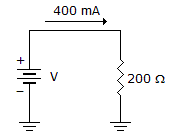# Electronics - Ohm's Law

### Exercise :: Ohm's Law - General Questions

11.What is the power in the given circuit?

 A. 32 W B. 80 W C. 500 W D. 16 kW

Answer: Option A

Explanation:

No answer description available for this question. Let us discuss.

12.

Ohm's law describes the mathematical relationship between

 A. ohms, kilohms, and megohms B. resistor size and resistor value C. resistance, voltage, and current D. none of the above

Answer: Option C

Explanation:

No answer description available for this question. Let us discuss.

13.

Energy is defined as the ability to

 A. produce heat B. produce light C. produce sound D. All of the above

Answer: Option D

Explanation:

No answer description available for this question. Let us discuss.

14.

What electromotive force would cause 20 A of current to flow through a 500resistor?

 A. 0.04 V B. 2.5 V C. 25.0 V D. 10,000 V

Answer: Option D

Explanation:

No answer description available for this question. Let us discuss.

15.

The rate at which work is performed is called

 A. current B. energy C. power D. voltage

Answer: Option C

Explanation:

No answer description available for this question. Let us discuss.

#### Current Affairs 2021

Interview Questions and Answers